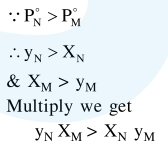# Liquid 'M' and liquid 'N' form an ideal solution.

Question:

Liquid 'M' and liquid 'N' form an ideal solution. The vapour pressures of pure liquids 'M' and ' $\mathrm{N}$ ' are 450 and $700 \mathrm{mmHg}$, respectively, at the same temperature. Then correct statement is:

$\left(x_{\mathrm{M}}=\right.$ Mole fraction of 'M' in solution ;

$x_{\mathrm{N}}=$ Mole fraction of 'N' in solution ;

$y_{\mathrm{M}}=$ Mole fraction of 'M' in vapour phase ;

$y_{\mathrm{N}}=$ Mole fraction of ' $\mathrm{N}$ ' in vapour phase)

1. $\left(x_{\mathrm{M}}-y_{\mathrm{M}}\right)<\left(x_{\mathrm{N}}-y_{\mathrm{N}}\right)$

2. $\frac{x_{M}}{x_{N}}<\frac{y_{M}}{y_{N}}$

3. $\frac{\mathrm{x}_{\mathrm{M}}}{\mathrm{x}_{\mathrm{N}}}>\frac{\mathrm{y}_{\mathrm{M}}}{\mathrm{y}_{\mathrm{N}}}$

4. $\frac{x_{M}}{x_{N}}=\frac{y_{M}}{y_{N}}$

Correct Option: , 3

Solution: